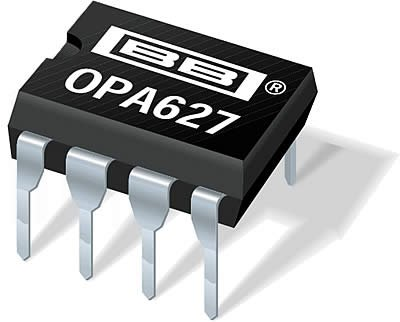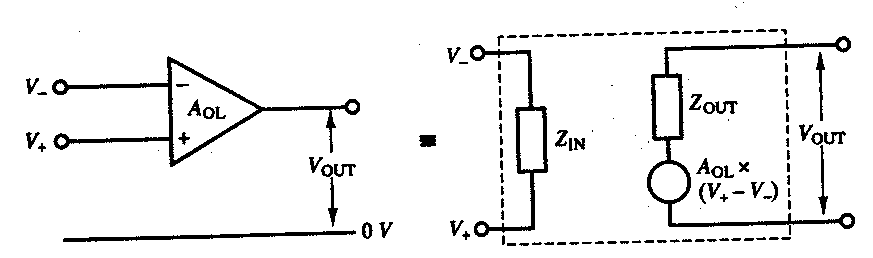# Briefly Discuss About Op amp(Operational Amplifiers)

## Definition of OP-AMP-:

An operational amplifier, or OP-AMP, is a high-gain electronic voltage amplifier & which can be used AC as well as DC both cases. Op amp  is DC coupled and contains a differential input and, usually, a single-ended output.It is basically designed to perform mathematical operations like that Addition, Subtraction, Multiplication, Division etc. OP-AMPs may be single, dual, quad etc. An Op amp produces an output voltage which is often several times larger than the voltage difference between its input terminals.

### Circuit Symbol Of OP-AMP## Practical Op amp (IC 741)

Open-loop gain G = vout / vinInfinite(∞)106
Input resistance RinInfinite(∞)1 MΩ – 2 MΩ
output resistance Rout050Ω – 100Ω
Offset voltage0<10 mV
Offset current010 nA
Open loop bandwidthInfinite(∞)≅1 MHz
Common-mode rejection ratio (CMRR)Infinite(∞)90 dB
Slew rateInfinite(∞)1 V/µSec
Noise0
Phase shift0